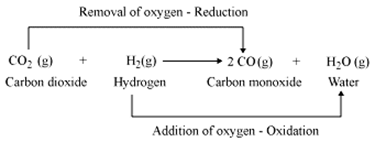### Question 2. Write answers to the following. What is the reaction called when oxidation and reduction take place simultaneously? Explain with one example.

Question 2.

Write answers to the following.

What is the reaction called when oxidation and reduction take place simultaneously? Explain with one example.

Answer:

When oxidation and reduction takes place simultaneously, the reaction is called redox reaction.

Oxidation: losing of electrons Reduction: gaining of electrons

Redox Reaction = Reduction + oxidation

For example:

CuO + H2→ Cu + H2O

i. In the reaction, CuO loses oxygen atom which means that reduction of CuO(copper oxide) takes place.

ii. H2 (hydrogen) takes up oxygen atom.

iii. As a result, formation of water takes place. This means hydrogen undergoes oxidation.

iv. Oxidation and reduction are taking place at the same time, thus it a redox reaction.

Another example: